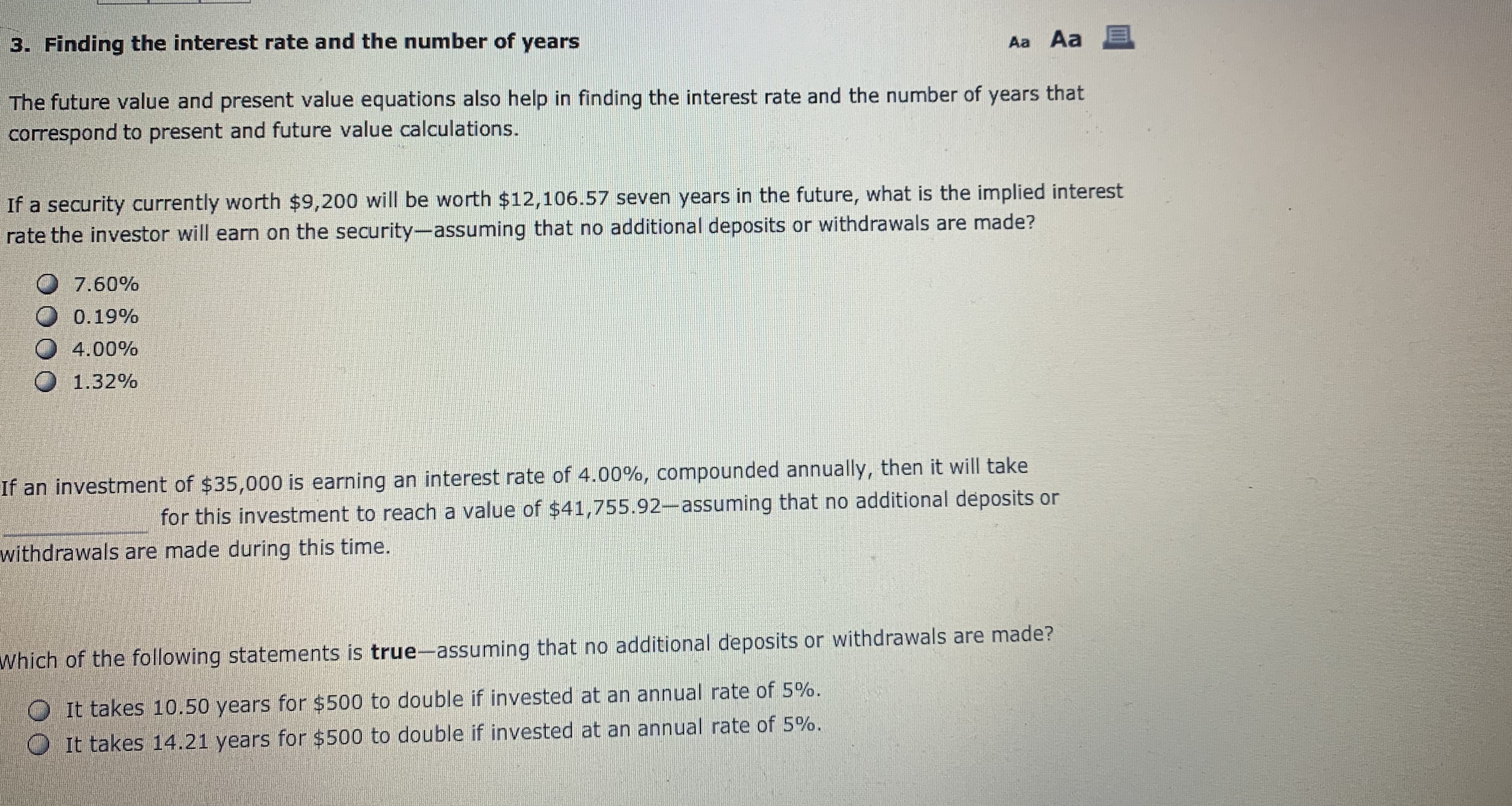# 3. Finding the interest rate and the number of yearsAa Aa EThe future value and present value equations also help in finding the interest rate and the number of years thatcorrespond to present and future value calculationsIf a security currently worth \$9,200 will be worth \$12,106.57 seven years in the future, what is the implied interestrate the investor will earn on the security-assuming that no additional deposits or withdrawals are made?7.60%0 0.19%О 4.00%О 1.32%If an investment of \$35,000 is earning an interest rate of 4.00%, compounded annually, then it will takefor this investment to reach a value of \$41,755.92-assuming that no additional deposits orwithdrawals are made during this time.Which of the following statements is true-assuming that no additional deposits or withdrawals are made?It takes 10.50 years for \$500 to double if invested at an annual rate of 5%.0 It takes 14.21 years for \$500 to double if invested at an annual rate of 5%.

Question
526 viewshelp_outlineImage Transcriptionclose3. Finding the interest rate and the number of years Aa Aa E The future value and present value equations also help in finding the interest rate and the number of years that correspond to present and future value calculations If a security currently worth \$9,200 will be worth \$12,106.57 seven years in the future, what is the implied interest rate the investor will earn on the security-assuming that no additional deposits or withdrawals are made? 7.60% 0 0.19% О 4.00% О 1.32% If an investment of \$35,000 is earning an interest rate of 4.00%, compounded annually, then it will take for this investment to reach a value of \$41,755.92-assuming that no additional deposits or withdrawals are made during this time. Which of the following statements is true-assuming that no additional deposits or withdrawals are made? It takes 10.50 years for \$500 to double if invested at an annual rate of 5%. 0 It takes 14.21 years for \$500 to double if invested at an annual rate of 5%. fullscreen
check_circle

star
star
star
star
star
1 Rating
Step 1

Calculating the implied interest rate the investor will earn on the security by using the formula of future value of money. We have,

Future value of money = C0 (1+r)n

Here,

C0 = Cash flow at period 0 = \$ 9,200

Future value of money = \$ 12,106.57

r = Rate of interest =?

n = Number of periods = 7 years

By substituting these value in the above formula. We get,

\$ 12,106.57 = \$ 9,200 (1+ r)7

(1+r)7 = \$ 12,106.57 / \$ 9,200

(1 +r)7 = 1.31593152173

(1+r) = (1.31593152173)1/7

(1+r) = 1.0400

r = 1.0400 – 1

r = 0.0400*100

r = 4.00 %

Therefore, the implied rate of interest the investor will earn on the security shall be 4.00%.

Step 2

Calculating the number of periods in which the investor will earn on the security by using the formula of future value of money. We have,

Future value of money = C0 (1+r)n

Here,

C0 = Cash flow at period 0 = \$ 35,000

Future value of money = \$ 41,755.92

r = Rate of interest =4.00 %

n = Number of periods =?

By substituting these value in the above formula. We get,

\$ 41,755.92 = \$ 35,000 (1.04)n

(1.04)n = \$ 41,755.92 / \$ 35,000

(1.04)n = 1.19302628571

(1.04)n = (1.04)4.5

n = 4.5 years

Therefore, the number of periods in which the investor will earn on the security shall be 4.5 years.

Step 3

Calculating the future value of money. We have,

Future value of money = C0 (1+r)n

Here,

C0 = Cash flow at period 0 = \$ 500

Future value of money =?

r = Rate of interest =5.00 %

n = Number of periods = 10.50 years

By substituting these value in the above f...

### Want to see the full answer?

See Solution

#### Want to see this answer and more?

Solutions are written by subject experts who are available 24/7. Questions are typically answered within 1 hour.*

See Solution
*Response times may vary by subject and question.
Tagged in